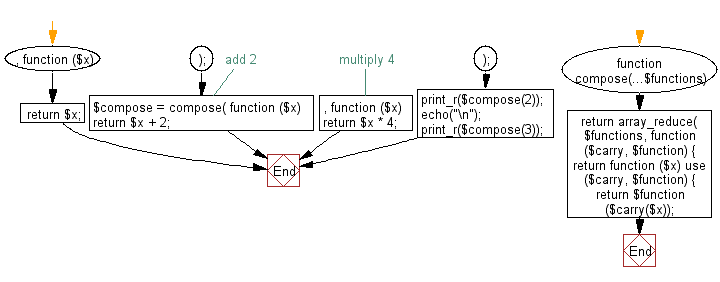﻿ PHP Exercise: Create a new function that composes multiple functions into a single callable - w3resource

# PHP Exercises: Create a new function that composes multiple functions into a single callable

## PHP: Exercise-98 with Solution

Write a PHP program to create a new function that composes multiple functions into a single callable.

Sample Solution: -

PHP Code:

``````<?php
//Licence: https://bit.ly/2CFA5XY
function compose(...\$functions)
{
return array_reduce(
\$functions,
function (\$carry, \$function) {
return function (\$x) use (\$carry, \$function) {
return \$function(\$carry(\$x));
};
},
function (\$x) {
return \$x;
}
);
}
\$compose = compose(
function (\$x) {
return \$x + 2;
},
// multiply 4
function (\$x) {
return \$x * 4;
}
);
print_r(\$compose(2));
echo("\n");
print_r(\$compose(3));

?>
```
```

Sample Output:

```16
20
```

Flowchart:PHP Code Editor:

Have another way to solve this solution? Contribute your code (and comments) through Disqus.

What is the difficulty level of this exercise?

Test your Programming skills with w3resource's quiz.

﻿

## PHP: Tips of the Day

Mutates the original array to filter out the values specified

Example:

```<?php
function tips_pull(&\$items, ...\$params)
{
\$items = array_values(array_diff(\$items, \$params));
return \$items;
}

\$items = ['x', 'y', 'z', 'x', 'y', 'z'];
print_r(tips_pull(\$items, 'y', 'z'));
?>
```

Output:

```Array
(
 => x
 => x
)
```